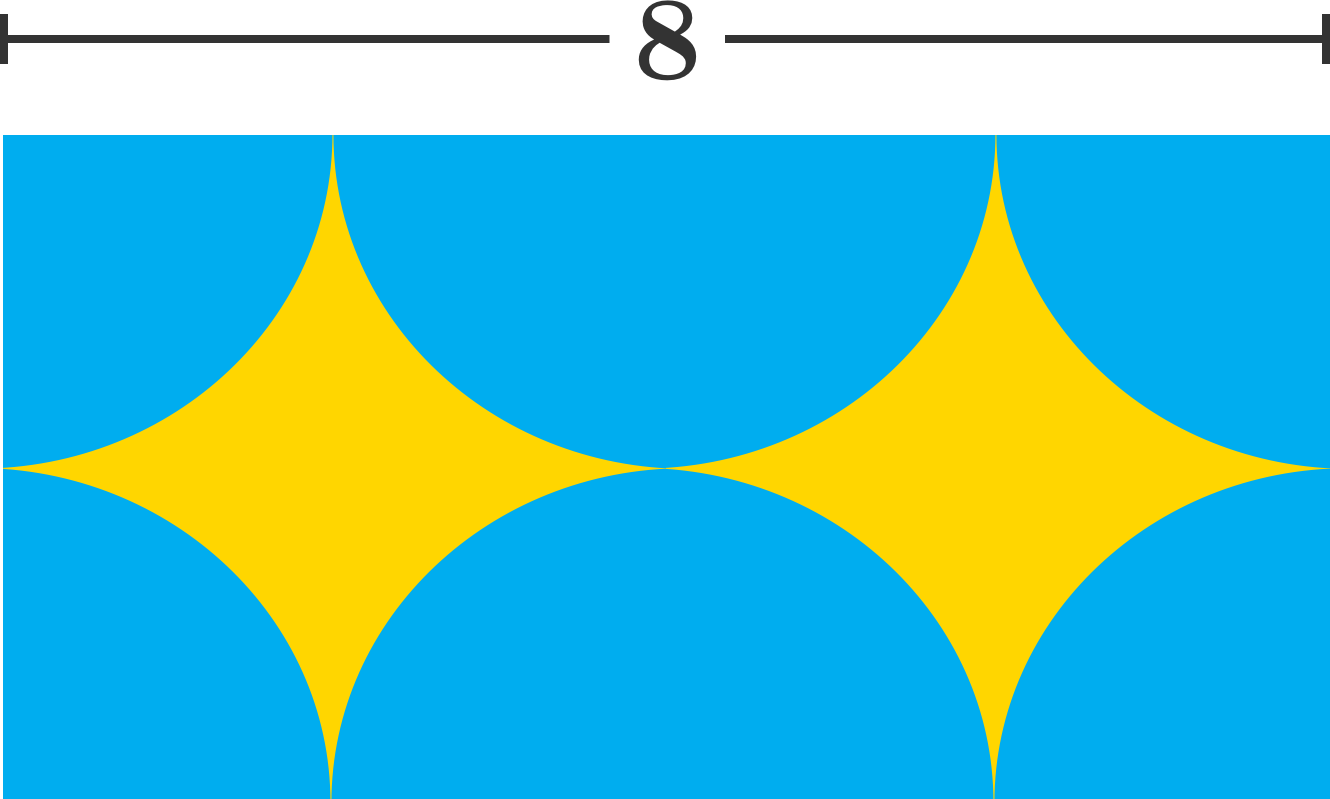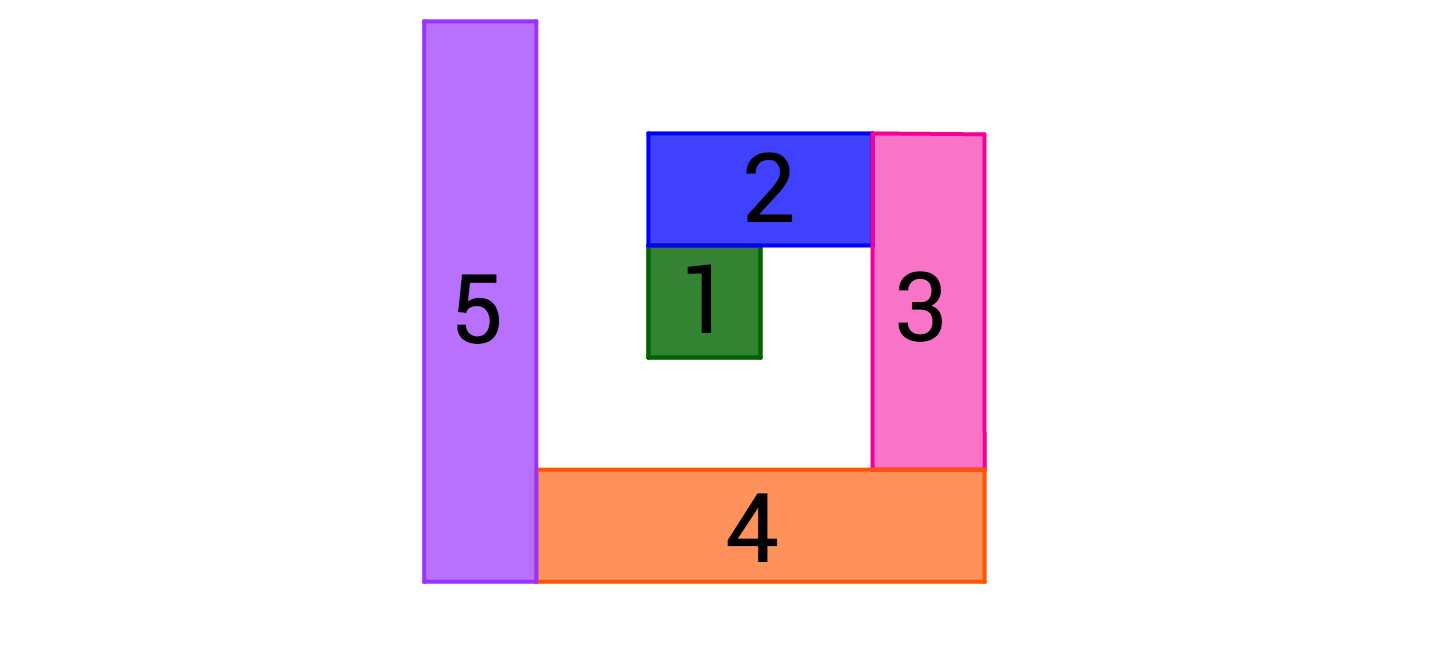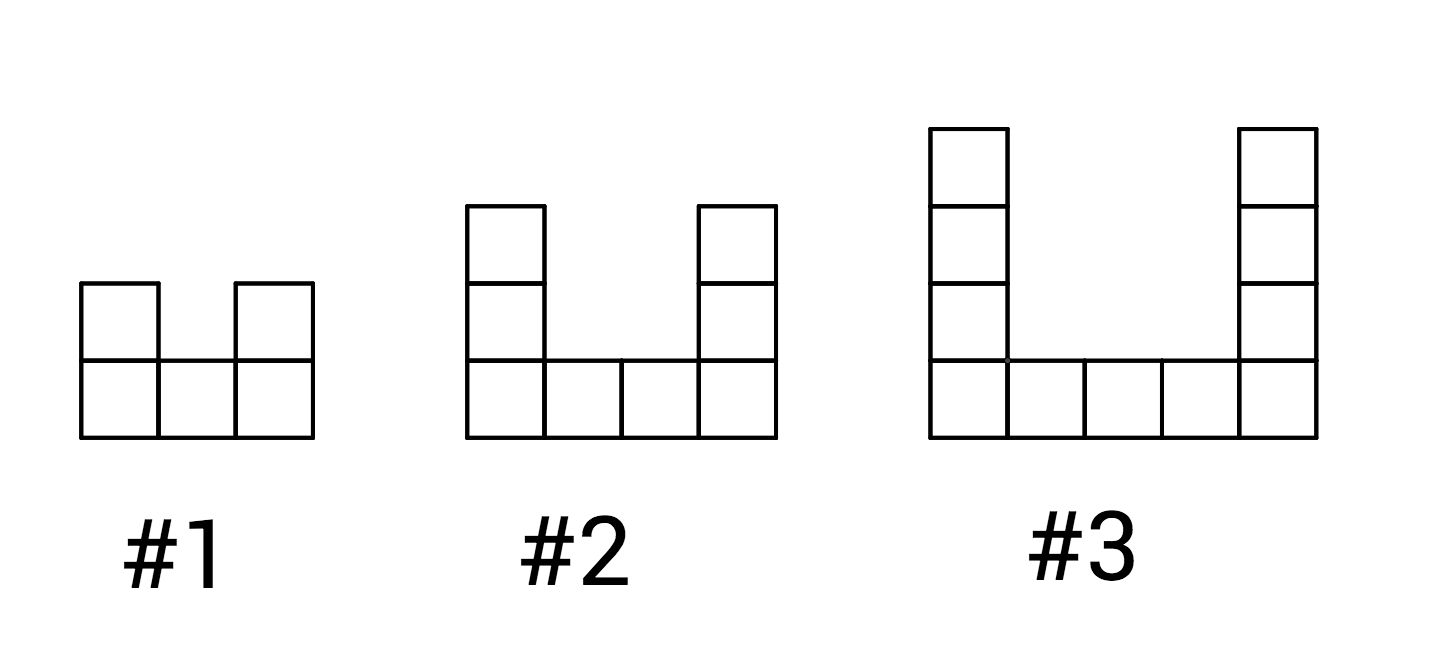Geometry

# Length and Area Warmup

If each of the side lengths of a triangle triples, then the perimeter will:

Is this statement true or false?

If the length and width of a rectangle double, then the area will double.If all circular pieces shown are semicircles and quarter circles, what is the area of the yellow region?Bo draws a 1-by-1 square (green). He begins a spiral by adding on a 2-by-1 rectangle (blue), then a 3-by-1 rectangle (pink), etc. Each rectangle is one unit longer than the previous rectangle added. What will be the perimeter of the spiral after the 8th rectangle is added?If the perimeter of Figure #1 is 12, what is the perimeter of the Figure #10?

×